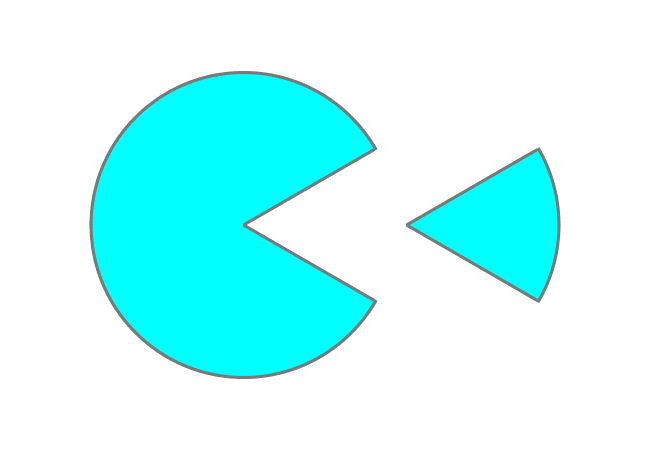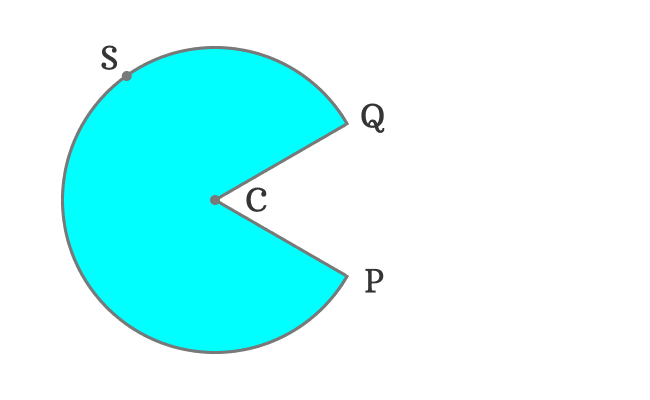Sector of a circleDefinition

A part of the circle enclosed by an arc and two radii is called a sector of the circle.

The intersection of two arcs and two radii divides the entire circle into two parts and each part is known as a sector of the circle.

Classification

On the basis of the amount of area enclosed by an arc and two radii, a circle is divided into two types of sectors.

1. Minor sector
2. Major sector

Example$C$ is a centre of a circle. The circumference is cut into two parts at points $P$ and $Q$. It formed two arcs. $R$ is a point on the minor arc and $S$ is a point on the major arc.

The interaction of two radii with the points $P$ and $Q$ split the entire circle into two parts and each part is known as a sector of the circle.

The two sectors are classified into minor and major sectors on the basis of the area.

1
Minor sector$\overline{CP}$ and $\overline{CQ}$ are two radii of the circle and $\stackrel{\Huge ⌢}{PRQ}$ is an arc of the circle.

The $arc \, PRQ$ is a minor arc. So, the sector formed by the radii $\overline{CP}$ and $\overline{CQ}$ and $\stackrel{\Huge ⌢}{PRQ}$ is a minor sector of the circle.

2
Major sector$\overline{CP}$ and $\overline{CQ}$ are two radii of the circle and $\stackrel{\Huge ⌢}{PSQ}$ is an arc of the circle.

The $arc \, PSQ$ is a major arc. Therefore, the sector formed by the radii $\overline{CP}$ and $\overline{CQ}$ and $\stackrel{\Huge ⌢}{PSQ}$ is a major sector of the circle.

Representation

A sector of the circle is represented in mathematics by combining centre, two endpoints of the arc and any point on the arc.

In this example, $C$ is the centre and $P$ and $Q$ are endpoints of the both arcs but $R$ is a point on the minor arc and $S$ is a point on the major arc.

So, the minor sector of the circle is represented as $sector \, CPRQ$ and major sector is denoted as $sector \, CPSQ$.

Latest Math Topics
Latest Math Problems
Email subscription
Math Doubts is a best place to learn mathematics and from basics to advanced scientific level for students, teachers and researchers. Know more